### An Introduction to Center of Mass and Collisions

2 Quick Videos to get you started

# Centre of Mass

The concept of centre of mass is inherent in the sense that it is applicable in a huge variety of areas, stretching from mass, gravitational science and electrostatics. Hence, the knowledge of such a topic is very important.

Conceptually, the point where the whole mass of body or system can be assumed to be concentrated for simplified study of its motion is called the centre of mass.

The centre of mass of an object is the single point that moves in the same way as a point mass having mass equal to the object would move when subjected to the same external forces that acts on the object. That is, if the resultant force acting on an object (or system of objects) of mass m is F->, the acceleration of the centre of mass of the object (or system) is given by .

a->cm = F->/m .

One should also understand that any point having an acceleration = F->/m, does not necessarily have to be the centre of mass i.e., it is a necessary but not sufficient condition.

If the system is considered to be composed of tiny masses m1, m2, m3, ..., at co-ordinates (x1, y1, z1), (x2, y2, z2), ..., then the co-ordinates of the centre of mass are given by

xcm = ∑ ximi /∑ mi,         ycm = ∑ yimi /∑ mi,         zcm = ∑ zimi /∑ mi

where the summations extend over all masses composing the object. Similarly, x-coordinate of centre of gravity is defined as Xcg = ∑ ximi /∑ mi. In a uniform gravitational field, the centre of mass and the centre of gravity coincide.

The position vector rcm of the centre of mass can be expressed in terms of the position vectors r1, r2, ... of the particles as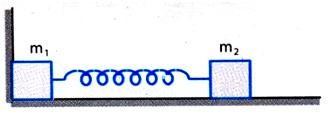In statistical language, the location of centre of mass is a mass-weighted average of the locations of all particles.

For a discrete system of particles the position (see Figure given below) of the centre of mass is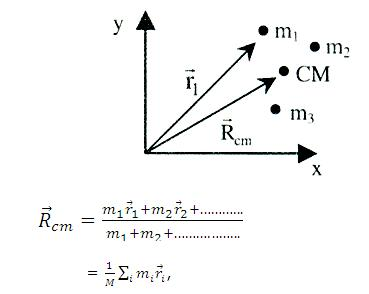where M = ∑imi and, ri = position vector of particle mi

So, the co-ordinates of centre of mass in three-dimensional Cartesian system will be,

Xcm = 1/M ∑imixi , Ycm = 1/M ∑imiyi ,

Zcm = 1/M ∑imizi,

# The centre of mass after removal of a part of a body

If a portion of a body is taken out, the remaining portion may be considered as, original mass (M) -mass of the removed part (m)

= {Original mass (M)} + {- mass of the removed part (m)}

The formula changes to:

Xcm = (Mx-mx')/(M-m) and Ycm = (My-my')/(M-m)

where primed ones represent the coordinate of the C.M. of the removed part.

## Centre of Mass for a Continuous Distribution

For a continuous distribution of mass, we can treat an element of mass dm at any position as a point mass and replace the summation by integration as shown below:

R->cm = 1/M ∫ x dm

So, we get Xcm = 1/M ∫ x dm

Ycm = 1/M ∫ y dm

Zcm = 1/M ∫ z dm,

# Types of Collision

It is the case when the velocity at the point of contact coincides with the common normal (line of impact).

2.      Oblique collision

It is the case when the velocities at the point of contact coincide with the line of impact.

## Newton's Experimental Law

Coefficient of restitution,

e = -  relative velocity of points of contact along the common normal after impact

relative velocity of points of contact along the common normal before impact

On the basis of coefficient of restitution, collision is divided into three types.

If e = 1, collision is called elastic if in this collision mechanical energy of the whole system is conserved.

If 0 < e < 1, collision is called inelastic.

If e = 0, collision is called perfectly inelastic.

# Velocities of colliding bodies after collision

In the following discussion, we will assume all the movements and velocities along a straight line. Let there be two bodies with masses m1 and m2 moving with velocities u1 and u2. They collide at an instant and acquire velocities v1 and v2 after collision. For sake of feasibility we assume that u1 > u2. Let the coefficient of restitution of the colliding bodies be 'e'. Then, applying Newton's experimental law and the law of conservation of momentum, we can find the value of velocities v1 and v2.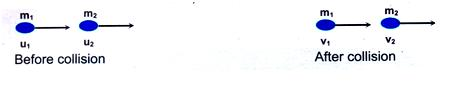Conserving momentum of the colliding bodies before and after the collision,

m1u1 + m2u2 = m1v1 + m2v2                                  ...... (i)

Applying Newton's experimental law, we have

v2 - v1 / u2 - u1 = -e

v2 = v1 - e (u2 - u1)                                             ...... (ii)

Putting (ii) in (i), we obtain

m1u1 + m2u2 = m1v1 + m2{v1 - e (u2 - u1)}

v1 = u1 (m1 - em2)/m1+m2  +  u2  (m2(1+e))/m1+m2     ...... (iii)

From (ii),

v2 = v1 - e (u2 - u1)

= u1m1(1+e)/m1+m2  +  u2  (m2-m1e)/m1+m2               ...... (iv)

When the collision is elastic; e = 1

Finally,       v1 = ( m1+m2/m1-m2 ) u1  +  ( 2m2/m1+m2 ) u2

and           v2 = ( 2m1/m1+m2 ) u1  +  ( m2+m1/m1-m2 ) u2

(i)     If m1 = m2, then

v1 = u2 and v2 = u1

When the two bodies of equal mass collide head on elastically, their velocities are mutually exchanged.

(ii)    If m1 = m2 and u2 = 0 then

v1 = -u1 and v2 = 0

Impulse

If a very large force acts on a body for a very short interval of time, then product of the force and the time is known as impulse. Impulse is a vector quantity.

I-> = ∫ F-> dt = ∫ (dp->)/dt dt = ∆p->

Hence, impulse is equal to the change in linear momentum p->.

Let us observe the collision of two marble balls A and B to understand the concept of impulse.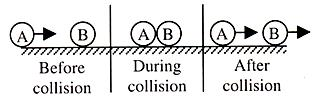During collision, the marble A is in contact with B for an interval that is quite sort in comparison to the time during which we are watching the ball and in this period, ball, A exerts a large force on B which is called impulsive force. Both the balls are deformed during the collision. This force varies with time. The impulsive force acting between the balls is given by

F->  =  dP-> / dt

=>       dP->  =  F->dt

Here dP-> is the change in momentum in time dt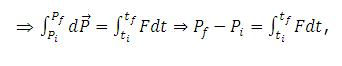,

Where Pf is the final momentum of the body at time tf and Pi is the initial momentum of the body at time ti.

The integral of a force over the time interval during which the force acts is called the impulse of the force. It is equal to the change in momentum of the body acted on by the impulsive force.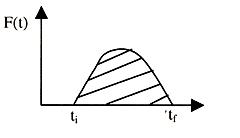The impulse of this force, ∫ti tf Fdt, is represented in magnitude by the area

under force-time curve, as shown in figure given above.

# A Few Easy Problems:

1) A wooden plank of mass m0 is resting on a smooth horizontal floor. A man of mass am0 starts moving from one end of plank to other. The length of plank is l0. Find the displacement of plank over the floor when man reaches other end of plank?

Solution: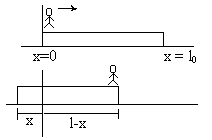The center of mass remains stationary as no external force. Hence,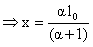2) A particle of mass 5kg is initially at rest. A force starts acting on it one direction whose magnitude changes with time. The force time graph is as shown in figure. Find the velocity of particle after 10 sec?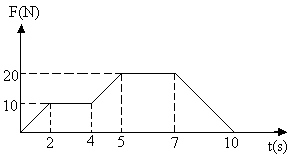Solution:

Impulse = DMomentum

= Area under F-t graph.3) Three identical balls, ball A, ball B, ball C are placed on a smooth floor on a straight line at the separation of 20m between balls as shown in figure. Initially balls are stationary. Ball A is given a velocity of 5m/s towards Ball B. Collision between A+B has coefficient of restitution as ½. But collision between B+C has coefficient of restitution as 1. What is the time interval between 2 consecutive collision between ball A and ball B?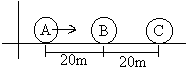Solution:

(1) Collision of A and B:

If velocities of ball A and ball B are V1 and V2 after collision then,

ThenAnd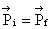(2) Collision between B and C:

Velocities get interchanged and so B is at rest after collision.

So time interval == 16 sec

4) A light spring of spring constant K0 is kept compressed with compression =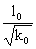between two masses of mass ‘m’ and ‘am’. When released the blocks acquire a velocity in opposite directions. The spring loses contact with both blocks when it comes in natural length! Find the final velocities of the two blocks?

Solution: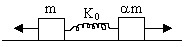Since no external force is acting, henceBut initial momentum = 0. Hence if final velocities of m and am are V1 and V2then,

mV1 - amV2 = 0

V1 = aV2 ------ (1)

Now energy remains conserved so,

Ei = Ef.5) A bullet of mass m0 strikes a block of mass ‘hm0’ with a speed of V0 and gets embedded into the mass ‘hm0’. The block is attached to a spring of stiffness ‘K’. Find the loss of K.E. of system after impact?

Solution: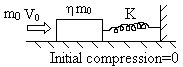As the spring force is a non impulsive force, so we can conserve momentum as:6) A stationary body explodes into four identical fragments such that three of them fly off mutually perpendicular to each other with same K.E =E0 Find energy of explosion?

Solution:

Let the three fragments move along x, y, z direction with velocities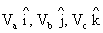.

Now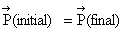# More Challenging Problems with Solutions

Example 1

If linear density of a rod of length L varies as l = A + Bx, compute its centre of mass.

Solution:

Because of symmetry of the body, we can consider a co-ordinate system as below for simplification.

Let x-axis be along the length of the rod and origin at one of its ends.

Obviously, Ycm = 0, Zcm = 0. Let us now find the x-coordinate.

If we take an element of the rod, of length dx at a distance x from origin.

dm = λdx = [A + Bx]dx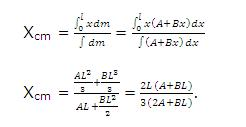Example 2

Find the centre of mass of a uniform semicircular arc of radius r.

Solution:

Let mass of the arc = M,

Radius of the arc = r,

\ Mass per unit length = M/πr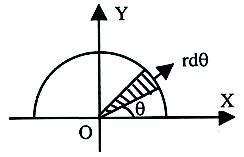Let us consider a small element rdθ,

\ Mass of small element = M/πr rdθ = M/π dθ.

Here Xcm = 0 (Think)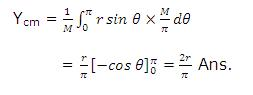Example 3

A block of mass M with a semicircular track of radius R rests on a horizontal frictionless surface. A uniform cylinder of radius r and mass m is released from rest at the top A (See Figure given below.). The cylinder slips in a semicircular frictionless track. (a) How far will the block move when the cylinder reaches the bottom (point B) of the track? (b) How fast is the block moving when the cylinder reaches the bottom of the track.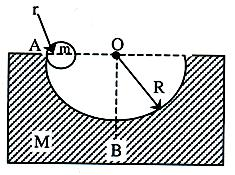Solution:

Given that initially the system is at rest so vcm-> = 0. Now in slipping as no horizontal force is applied, the horizontal component of velocity of centre of mass of the system must remain constant. So, if v-> and V-> are the velocities of cylinder and block at B relative to the ground

(mv-> + MV->)/(m+M) = const. = 0

i.e. mv-> + MV-> = 0                 as m + M = finite                ...... (1)

or     m (∆r1->)/∆t+(∆r2->)/∆t M=0             [asv-> = (∆r1->)/∆t]

or mΔr1-> + MΔr2-> = 0             as Δr1-> = d->

or md1 - Md2 = 0                as d2-> is opposite to d1->           ....... (2)

Now, when the cylinder reaches from A and B, it is displaced by a distance (R - r) horizontally to the right relative to the block. So, if the displacement of the block relative to ground is d2 to the left, the displacement of cylinder relative to ground to the right will be

d1 = (R-r) - d2                                                    ......(3)

Substituting d1 from Equation (3) in(2), we get

M[(R-r)-d2]-md2 = 0

i.e. d2 = (m(R-r))/(M+m) (to the left)

(b)    Applying conservation of ME between point A and B

mg (R-r) = 1/2 mv2 + 1/2 MV2

Substituting the value v from Equation (1) in it

mg (R - r) = 1/2,   (-M/m V)2 + 1/2 MV2

Which on simplification gives

V = m √((2g(R-r))/(M(m+M)))            (to the left).               Ans.

Example 4

A ball of mass 1 kg collides with another ball of mass 1 kg at an angle 30o with the x-axis with a velocity of 1 m/s. Find the final velocities if after collision both the masses go at 45o as shown in the Figure given below.

Solution: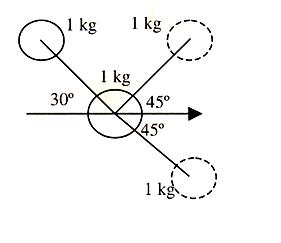Initial momentum (along x axis) = (1×1) cos 30o

= √3/2 kgm/s

Initial momentum (along y-axis) = (1×1) sin 30o + 0

= 1/2 kgm/s

Final momentum (along x-axis) = 1 × v1 cos 45o + × v2 cos 45o.

= (v1 + v2) 1/√2

Final momentum (along y-axis) = v1 sin 45o - v2 sin 45o

= (v1 - v2) 1/√2

Since no external force acts along x-axis or y-axis

=> √3/2 (v1 + v2) 1/√2   =>   v1 + v2 = √3/2 ............ (1)

& 1/2 = (v1 - v2) 1/√2   => v1 - v2 = 1/√2 ...... (2)

Solving (1) & (2) we get

v1 = [(√3+1)/(2√2)]m/s

v2 = [(√3-1)/(2√2)]m/s

Example 5

A ball of mass m moving with a speed 'u' collides elastically with another identical stationary balls.

(a)    Find the velocities of the balls after collision if the impact is direct.

(b)    Find the angle between velocities after collision if they collide obliquely.

Solution:

(a)    Let v1 and v2 be the final velocities of balls.

Conservation of momentum

mu = mv1 + mv2

Newton's experimental law

v1 - v = -(u - 0)

Hence u = v1 + v2 and -u = v1 - v2

=> v1 = 0 and v2 = u.

Hence colliding ball becomes stationary and transfers all of its speed to the other ball.

(b)    mu = mv1 cos θ1 + mv2 cos θ2                               (i)

0 = mv1 sin θ + mv2 sin θ2                                    (ii)

(1/2) mu2 = (1/2) mv12 +  (1/2) mv22                     (iii)

Squaring and adding (i) & (ii)

u2 = v12 + v22 + 2v1v2 cos (θ1 + θ2)

Using (iii), we have

u2 = v12 +  v22

Then, cos (θ1 + θ2) = 0

or     θ1 + θ2 = 90o

Hence, the balls move at right angles to each other after collision.

Example 6

A bullet of mass m moving with a horizontal velocity u strikes a stationary block of mass M suspended by a string of length L. The bullet gets embedded in the block. What is the maximum angle made by the string after impact?

Solution:

Let v be the combined velocity of the bullet-block system just after collision. Then, by conservation of linear momentu.

mu = (m + M)v                                          (1)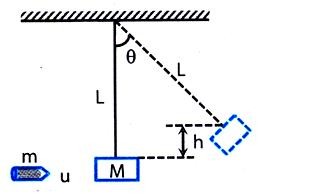After collision, the K.E. of the bullet-block system gets converted into potential energy.

\        (m + M)v2 = (m + M)gh

or     v = √2gh

Putting this value in equation (1), mu = (m + M) √2gh

or     h =  u2/2g [m/(m+M)]2

From the figure, cos  = L-h / L = 1 - h/L

\                                     = cos-1 [1-u2/2gh (m/(m+M))2 ]